# HBSE 7th Class Maths Solutions Chapter 8 Comparing Quantities Ex 8.2

Haryana State Board HBSE 7th Class Maths Solutions Chapter 8 Comparing Quantities Ex 8.2 Textbook Exercise Questions and Answers.

## Haryana Board 7th Class Maths Solutions Chapter 8 Comparing Quantities Exercise 8.2

Question 1.
Convert the given fractional number to per cents.
(a) $$\frac{1}{8}$$
(b) $$\frac{5}{4}$$
(c) $$\frac{3}{40}$$
(d) $$\frac{2}{7}$$
Solution: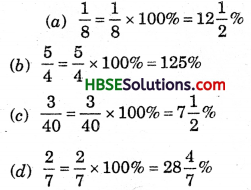Question 2.
Convert the given decimal fractions to per cents.
(a) 0.65
(b) 2.1
(c) 0.02
(d) 12.35
Solution: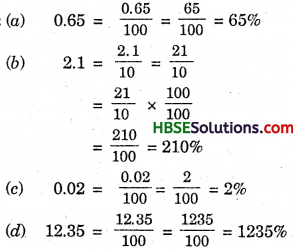Question 3.
Estimate what part of the figures is coloured and hence find the per cent which is coloured.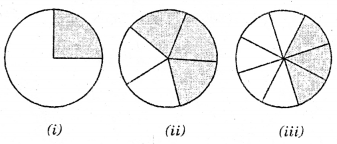Solution:
(j) Percentage of coloured part
= $$\frac{1}{4}$$ × 100% = 25%
(ii) Percentage of coloured part
= $$\frac{3}{5}$$ × 100% = 60%
(iii) Percentage of coloured part
= $$\frac{3}{8}$$ × 100% = 37$$\frac{1}{2}$$%

Question 4.
Find :
(а) 15% of 250
(b) 25% of 150
(c) 1% of 1 hour
(d) 20% of Rs. 2500
(e) 75% of 1 kg.
Solution: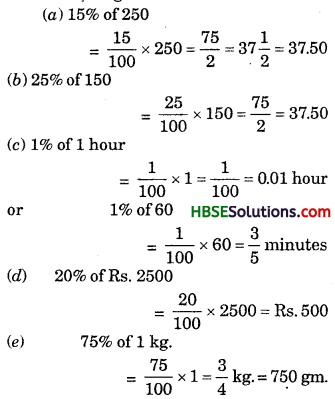Question 5.
Find the whole quantity if:
(a) 5% of it is 600
(b) 12% of it is Rs. 1080
(c) 40% of it is 500 km.
(d) 70% of it is 14 mins.
Solution:
(a) Hence, 5% of x = 600
$$\frac{5}{100}$$ × x — 600
or $$\frac{x}{20}=\frac{600}{1}$$
or, x = 20 × 600
∴ x = 12000.(b) 12% of x = 1080
or, $$\frac{12}{100}$$ × x = 1080
or, 12x = 1080 × 100
or, x = $$\frac{1080 \times 100}{12}$$
∴ x = Rs. 9000

(c) 40% of x = 500
or, $$\frac{40}{100}$$ × x = 50
or, 2x = 5 × 500
or x = $$\frac{5 \times 500}{2}$$
100 = 500
or, 2x = 5 × 500
or, x = $$\frac{5 \times 500}{2}$$
∴ x = 1250 km.

(d) 70% of x = 14 mins.
or, $$\frac{70}{100}$$ × x = 14
or, 7x = 14 × 10
or x = $$\frac{14 \times 10}{7}$$
x = 20 mins.

(e) 8% of × 8 = 40 litres 40
or, $$\frac{8}{100}$$ × x = 40
or, 2x = 40 × 25
or, x = $$\frac{40 \times 25}{2}$$
x = 500 litres.

Question 6.
Convert given per cents to decimals and also to fractions in simplest forms :
(a) 25%
(b) 150%
(c) 20%
(d) 5%
Solution: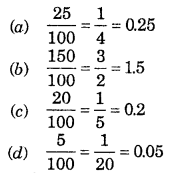Question 7.
In a city, 30% are females, 40% are males and remaining are children. What per cent are children ?
Solution:
Percent of children
= [100 – (30 + 40)]%
= (100 – 70)% = 30%

Question 8.
Out of 15,000 voters in a constituency, 60% voted. Find the percentage of voters who did not vote. Can you now find how many actually did not vote?
Solution:
Percent of vote = 60%
Percent of not vote = (100 – 60)%
= 40%
No. of actually did not vote
= 40% of 15000 = $$\frac{40}{100}$$ × 15000 = 6000

Question 9.
Meeta saves Rs. 400 from her salary. If this is 10% of her salary. What is her salary ?
Solution:
Let her salary be Rs. x.
Hence, 10% of x = 400
or $$\frac{10}{100}$$ × x = 400
or x = 400 × 10
= Rs. 4000Question 10.
A local cricket team played 20 matches in one season. It won 25% of them. How many matches did they win ?
Solution:
No. of total match = 20
percentage of won match = 25%
∴ Total number of winning match
= 20 of 25% = 20 × $$\frac{25}{100}$$ = 5